## Mathematics Paper 2 Questions and Answers - Bungoma Diocese Mock Exams 2021/2022

Instructions to candidates

• The paper contains TWO sections: Section I and Section II.
• Answer ALL the questions in Section I and any five questions from Section II
• Show all the steps in your calculations, giving your answers at each stage in the spaces below each question.
• Non – programmable silent electronic calculators and KNEC Mathematical tables may be used, except where stated otherwise.

SECTION 1 (50 MARKS)
Answer all the questions in this section

1. Use a calculator to find V if 1  1     −     1          (2mks)
V   23.9      38.45
2. Solve for X in Log (7X – 3) + 2 Log 5 = 2 + Log (X+3) (3mks)
3. A quantity P is partly constant and partly varies as the square of Q. when Q = 2, P = 40 and when Q = 3, P = 65. Determine the equation connecting P and Q (3mks)
4. Expand (1 − 1/2X)6 up to the fourth term; hence use your expansion to evaluate 0.9966 correct to 4 decimal places. (4mks)
5. Simplify √5 + 3 . Give the answer in the form of a + b√c where a, b and c are integers (3mks)
√5 − 2
6. Given that X−5, X−3 and 2X−3 are three consecutive terms of a geometric progression, find the possible values of X and the ratio (2X+1):(X+2) (4mks)
7. The figure below is a segment of a circle cut off by a chord AB. Line CD is a perpendicular bisector of chord AB.If AB is 24cm and CD is 8cm, calculate the radius of the circle. (3mks)
8. By completing the square, solve for x in the equation 2x2 − 6 = x. (3mks)
9. Given that y = b  − bx² make x the subject (3mks)
cx2 − a
10. The base and height of a right-angled triangle are 4cm and 5cm respectively. Calculate the percentage error in its area. (3mks)
11. Given that P =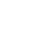, find ;
1. Its inverse (1mk)
2. The value of x and y if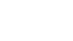(3mks)
12. The equation of a circle is given by x2 + y2 + 6x – 10y − 30 = 0. Determine the radius and center of the circle (3mks)
13. Find the value of X which satisfies the equation 52x − 6 × 5x + 5 = 0 (3mks)
14. A scooter mixes oil and petrol in the ratio 5:19. If petrol costs Ksh. 130 per liter and oil costs Ksh. 250 per liter, find the cost of a liter of the mixture. (2mks)
15. Solve the pair of equations simultaneously (4mks)
2x – y = 3
x2 – xy = −4
16. The cash price of a water pump is Ksh. 38,000. Mr. Ahero opts to buy the pump on hire purchase terms by paying a deposit of Ksh. 6,500 and 24 equal monthly installments. Calculate the amount of each installment, if simple interest of 20% p.a is charged. (3mks)

SECTION II (50 MARKS):
Attempt any five questions in this section

1. The first term of an arithmetic sequence is equal to the first term of the geometric sequence. The second term of the arithmetic sequence is equal to the fourth term of the geometric sequence, while the tenth term of the arithmetic sequence is equal to the seventh term of the geometric sequence.
1. Given that a is the first term and d is the common difference of the arithmetic sequence while r is the common ratio of the geometric sequence, write down two equations connecting the arithmetic and geometric sequences. (2mks)
2. Find the value of r that satisfies the geometric sequence (4mks)
3. Given that the tenth term of the geometric sequence is 5120, find the values of a and d (2mks)
4. Calculate the sum of the first 20 terms of the arithmetic sequence (2mks)
2. Three quantities R, S and T are such that R varies directly as S and inversely as the square of T.
1. Given that R = 480 when S = 150 and T = 5, write an equation connecting R, S and T (3mks)
2. Find,
1. the value of R when S = 160 and T = 1.6 (3mks)
2. the percentage change in R if S increases by 5% and T decreases by 20% (4mks)
3. The table below shows income tax rates
 Monthly income in Kenya shillings (Ksh) Tax rate % in each shilling Up to 9680 10% From 9681to18800 15% From 18801 to 27920 20% From 27921 to 37040 25% From 37041 and above 30%
In that year Okumu’s salary amounted to K£ 45,000 p.a and he received allowances totaling Ksh. 300,000 p.a. He was entitled to:-
1. Monthly personal relief of Ksh. 1,056
2. Monthly insurance relief at the rate of 15% of the premium paid
Okumu paid a monthly premium of Ksh. 2,500 towards his life insurance policy
Calculate
1. His gross monthly income in Ksh (2mks)
2. The monthly income tax he pays (5mks)
3. His net monthly income, if his other monthly deductions were: - Ksh. 4,800 to HELB, Ksh. 5,000 to his co-operative and Ksh. 2,800 towards a bank loan repayment. (3mks)
4. Square OABC with vertices O(0,0),A(2,0), B(2,2) and C(0,2) is mapped onto O’(0,0), A’(2,0), B’(5,2) and C’(3,2) by the matrix T =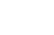1. Find T (3mks)
2. Draw O’A’B’C’ and reflect it on the line x + y = 0 to obtain O”A”B”C” (4mks)  (attach graph paper)
3. What single matrix P maps OABC to O”A”B”C” (3mks)
5. In the triangle PQR below L and M are points on PQ and QR respectively such that PL: LQ = 1:3 and QM: MR = 1:2, PM and RL intersect at X. Given that PQ = b and PR=c,1. Express the following vectors in terms of b and c
1. QR (1mk)
2. PM (1mk)
3. RL (1mk)
2. By taking PX = hPM and RX = kRL where h and k are constants find two expressions of PX in terms of h, k, b and c. Hence determine the values of the constants h and k. (6mks)
3. Determine the ratio LX : XR (1mk)
6. During a traffic crackdown, 1,000 motor cycles were sampled. 250 of these were found to lack necessary driving gear, 200 had no valid insurance and 300 lacked the driving license. Taking the sample to represent all motorcycles in the country;
1. Represent the information in a tree diagram (3mks)
2. Find the probability that, a motorcyclist at any given time
1. Has no driving license (3mks)
2. Lacks a valid insurance but is in proper driving gear and has a valid driving license (2mks)
3. Has none of the offence (2mks)
7. In the figure below, K L M and N are points on the circumference of a circle centre O. The points K, O, M and P are on a straight line. PQ is a tangent to the circle at N. Angle KOL = 130° and angle MKN = 40°Find the values of the following angles, stating the reasons in each case:
1. <MLN (2mks)
2. <OLN (2mks)
3. < LNP (2mks)
4. <MPQ (2mks)
5. <KNQ (2mks)
8. Complete the table below for y = Sin 2x and y = Sin(2x+30)° giving values to 2 d.p (1mk)
 x° 0° 15° 30° 45° 60° 75° 90° 105° 120° 135° 150° 165° 180° Sin 2x 0.00 0.87 −0.87 0.00 Sin (2x+30)° 0.50 0.50 −1.00 0.50
1. Draw the graph of y = Sin 2x and y = Sin(2x+30)° on the same axis (4mks)
2. Use your graph to solve Sin(2x+30)°− Sin 2x = 0 (1mk)
3. Describe the transformation which maps the wave Sin 2x onto the wave Sin (2x +30) (2mks)
4. State the amplitude and period of y = a cos (bx +c) (2mks)## MARKING SCHEME

NO   WORKING   MARKS   COMMENT
1  1/V= 0.01583
V =      1
0.01583
V = 63.17
M1
A1

2
2

Log (7X – 3) + 2 Log 5 – Log (X+3) = 2
Log 25(7X – 3)= 2
(X+3)
102 = 25(7X – 3)
(X+3)
100x +300 = 175x – 75
X =15

M1

M1

A1

3
3 P = k + cQ2
40 = k + 4c
65 = k + 9c
c = 5, k = 20
p = 20 + 5 Q2

M1
A1

B1

Both eqns
Both values
3
4  1(1/2X)+ 6(1/2X)+ 15(1/2X)2 + 20(1/2X)3
1 − 3 + 15   −  20
x     4x2     8x3
1 −   3   +  3   −      1
125   125    781250
= 0.9760

M1
M1

M1
A1

4
5

(√5+ 3) × (√5+ 2)
(√5- 2)     (√5+ 2)
5+2√5+3√5+6
5 - 4
11 + 5√ 5

M1

M1
A1

3
6  = X−3 = 2X−3
X−5     X−3
x2 − 6x +9 = 2x2 −13x +15
(x − 6)(x −1) = 0
x = 6 or x = 1
13:87 or 1:1

M1

M1
A1
B1

4
7122 = (r − 8)2 = r2
144 + r2 − 16r + 64 = r2
R= 13
M1

M1
A1

3
8  x2 – ½ x + (¼ )2 =3 + (¼ )2
(x – ¼ )2 = ± 49/16
x = 3/2 0r -4
M1
M1
A1

3
9  (cx2 – a) y = b – bx
cx2 + bx = b + ay
x2 = b+ ay
cy+b
x =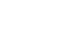M1
M1

A1
✓Formation of the equations
✓attempt to solve
3
10 Min area = ½ × 4.5 × 3.5 = 7.875

Max area = ½ × 5.5 × 4.5 = 12.375
Absolute Error = ½ (7.875 + 12.375)
Percentage Error = 8.4375 × 100% = 84.38%
10

M1

M1A1

3
11
1.  ½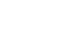2.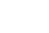(x/y) = (3/2)
½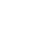(x/y) = ½(3/2)
½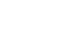(x/y) = ½ (6/-8)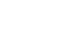(x/y) = (3/−4)
X = 3 , y = −4

M1

M1

M1

A1

4
12  x2 +6x + 9 + y2 – 10y +25 = 30 + 9 + 25
(x + 3)2 + (y – 5)2 = 82
Centre (-3, 5) Radius = 8 units
M1
M1
A1

13  Let 5x = y
y2 – 6y + 5 = 0
(y – 5) (y – 1) = 0
y = 5 or 1
5x = 5 or 5x = 1
x = 1 or x = 0

M1

A1

B1

3
14   (250 × 5) + (130 × 19) = 155
5+19
M1A1
2
15  y = 2x – 3
x2 – x(2x – 3) = – 4
(x – 4) (x – 1) = 0
x =4 or x = 1
y = 5 or y = –1
M1
M1
A1
B1

4
16  31500 + (31500 × 20/100 × 24/12 ) = 44,100
44100  = 1,837.50

24
M1
M1A1

3
17
1. a + d = ar3
a + 9d = ar6
2. r3 = a+d
a
a + 9d = a(a+d)2
a
7a = d
r3 = a+7a  = 8
a
r = 2
3. ar9 = 5120
a(29) = 5120
a = 10
d = 70
4. S20 = 20/2{2(10)  + 19×70}
= 10(20 + 1330)
= 13,500

B1
B1

M1

M1
M1

A1

M1

A1
M1

A1

a and d
10
18
1. 480 = 150 K
25
K = 802
R = 80S
T2
2.
1. R = 80 ×160
1.62
=5,000
2. R1 = 80 × 1.05S
(0.8T)2
S
R1 = 131.25 T2
Change in R = 51.25S
T2
% Change in R = 51.25 ×100%
80
= 64.0625%

M1
M1
A1

M1M1

A1

M1

M1

M1

10
19
1. (45,000×20)+(300,000)
12
= 100,000
2. 9,680 ×10% = 968
9,120 × 15% = 1,368
9,120 ×20% = 1,824
9,120 × 25% = 2,280
62,960 × 30% = 18,888
Total = 25,328
Net tax = 25, 328 – (1,056 + 15% of 2,500)
= 23,897
3. 100, 000 – (23,897 + 2,500 + 4,800 + 5,000 + 2,800)
= 61, 003

M1

A1

M1

M1

M1
M1
A1
MN1M1
A1

10
20
1.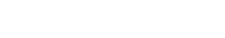a = 1      b = 1.5
c = 0       d = 1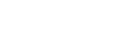2.3.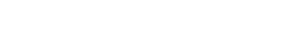w = 0      x = −1
y = −1     z = −1.5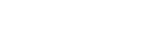21
1.
1. QR = c – b
2. PM = 1/3 (2b − c)
3. RL = ¼ b − c
2. PX =2/3 h b+ 1/3 h c
PX= ¼ k b + (1 – k) c
2/3 h b+ 1/3 h c = ¼ k b + (1 – k) c
h= 3/8 k
1/3 h = 1 – k
1/8k = 1 – k
k = 8/9
h = 1/3

B1
B1
B1

B1
B1
B1

B1
B1

B1

One unknown for both values
10
22
1.2.
1. (25/100 × 2/10 × 3/10)+(25/100 × 8/10 × 3/10)+(75/100 × 2/10 × 3/10) = (75/100 × 8/10 × 3/10)
3/200 + 3/50 + 9/200 + 9/50
= 3/10
2. (75/100 × 2/10 × 7/10) + (75/100 × 8/10 × 7/10)
= 21/100 + 21/5063/100
3. (75/100 × 8/10 × 7/10)
= 21/50

M1

M1
A1
M1
A1

M1
A1

23
1.  <MLN = 40° (angles subtended by the same arc NL are equal
2. <OLN = 25° (65° – 40°)
3. <LNP = 65° (40 + 25 angles subtended in alternate segment)
4. <MPQ = 10°(180°− (130+40))
5. <KNQ = 50° (Supplementary angles)

24
 x° 0° 15° 30° 45° 60° 75° 90° 105° 120° 135° 150° 165° 180° Sin 2x 0.00 0.05 0.87 1.00 0.87 0.50 0.00 −0.50 −0.87 −1.00 −0.87 −0.50 0.00 Sin (2x+30)° 0.50 0.87 1.00 0.87 0.50 0.00 −0.50 −0.87 −1.00 −0.87 −0.50 0.00 0.50
1.2. x = 37.5°, x = 127.5°
3. a translation of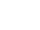4. amplitude a
Period 360/b

• ✔ To read offline at any time.
• ✔ To Print at your convenience
• ✔ Share Easily with Friends / Students